# 1.介绍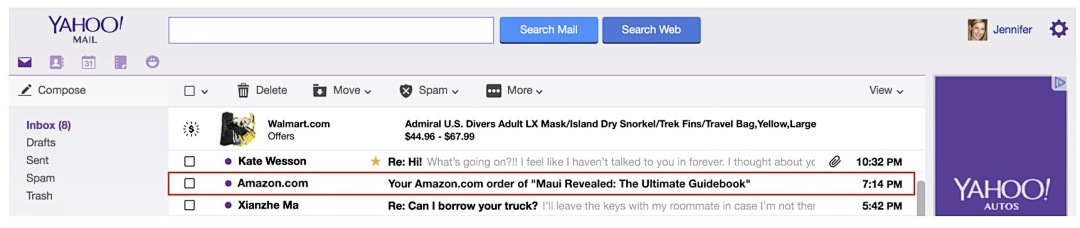# 3.方法

## 3.1 低维商品embeddings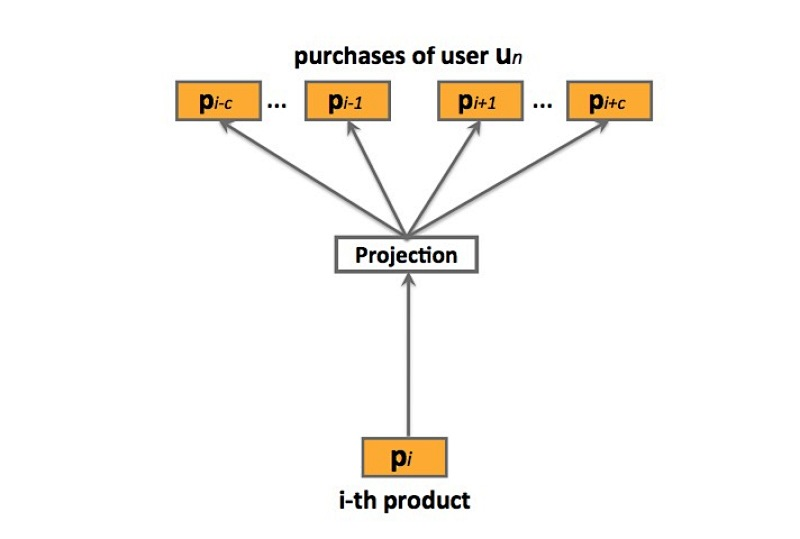prod2vec：prod2vec模型会将一个购买序列看成是一个“句子”，在这个序列中的商品看成是“词”。详见图2, 更特殊的，prod2vec使用skip-gram模型来学习商品表示，通过以下的最大化目标函数：

…(3.1)

…(3.2)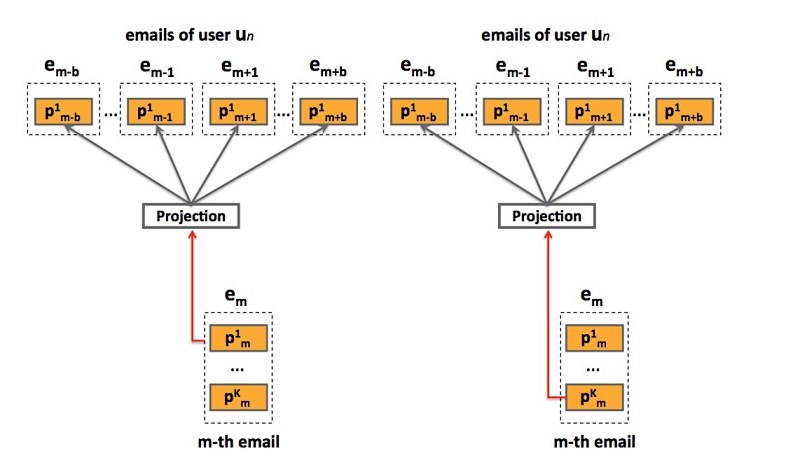bagged-prod2vec：为了对多个商品同时购买的行为做出解释，我们提出了一个修改版本的skip-gram模型，它引入了一个概念：购物袋（shopping bag）。如图3所示，该模型会在email receipts级别进行操作，而非在商品级别。通过对email序列s上的一个修改版目标函数进行最大化，来学习商品向量表示：

…(3.3)

learning：该模型使用SGA（ stochastic gradient ascent）进行最优化，很适合大规模问题。然而，在(3.1)和(3.3)中的梯度计算$\Delta L$，很适合词汇size P，实际任务中，计算开销会随着P的增大而变得很昂贵，很容易达到上百万的商品。另一方面，我们使用negative sampling方法，它能显著减小计算复杂度。

## 3.2 prod-2-prod预测模型

prod2vec-topK：给定一个购买商品，该方法会为所有在词汇表中的商品计算cosine相似度，并推荐最相似商品的top K

prod2vec-cluster：为了能做出更多样化(diverse)的推荐，我们考虑将相似商品分组成聚类，从与之前购买商品相关的聚类中推荐商品。我们应用K-means聚类算法来在hadoop FS上实现，将商品基于cosine相似度进行聚类。我们假设：在从聚类$c_i$上进行一个购买后、再从任意第C个聚类中进行一次购买的行为，符合一个多项式分布（multinomial distribution）$M_u(\theta_{i1}, \theta_{i2}, ..., \theta_{iC})$，其中$\theta_{ij}$是从聚类$c_i$中进行一次购买后、接着从聚类$c_j$中进行一次购买的概率。为了估计参数$\theta_{ij}$，对于每个i和j，我们采用一个最大似然方法：

$\hat {\theta_{ij}} = \frac{c_i购买后跟c_j的次数}{c_i购买的总数}$ …(3.4)

• count of ci purchases: c_i购买的数目
• # of times ci purchase was followed by cj: c_i购买后跟c_j的次数

## 3.3 User-to-product预测模型

user2vec：受paragraph2vec算法的启发，user2vec模型会同时学习商品和用户的特征表示，它会将用户当成是一个“全局上下文”。这样的模型如图4所示。训练数据集来自于用户购买序列S，它会包含$u_n$和其它已购商品（通过购买时间序排列），$u_n = (p_{n1}, p_{n2}, ..., p_{nU_n})$，其中$U_n$表示用户$u_n$购买的items数目。在训练期间，用户向量会被更新，来预测从他的email receipts中的商品，其中，学到的product vectors会预测在上下文中的其它商品。出于表示的简洁性，在下面，我们会表示no-bagged版本的模型，注意，使用bagged版本进行扩展也很方便。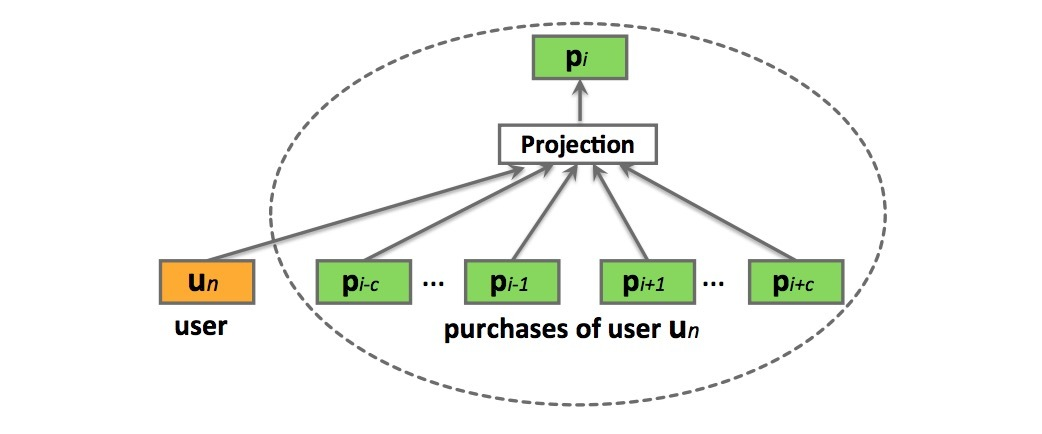…(3.5)

$P(p_{ni}|p_{n,i-c}: p_{n,i+c}, u_n) = \frac{e^{\bar{v}^T v_{p_{ni}}'}}{ \sum_{p=1}^{V} e^{\bar{v}^T v_p'}}$ …(3.6)

$\bar{v} = \frac{1}{2c+1} (v_{u_n} + \sum_{-c \leq j \leq c ,j \neq 0} v_{p_{n,i+j}})$ …(3.7)

$P(u_n | p_{n1}: p_{nU_n}) = \frac{e^{\bar{v}_n^T v_{u_n}'}} {\sum_{p=1}^V e^{\bar{v}_n^T v_p'}}$ …(3.8)

…(3.9)

user2vec模型的一个主要优点是，商品推荐是基于该用户的购买历史进行量身定制的。然而，缺点是，该需要需要非常频繁地进行更新，不同于product-to-product方法，它可以长期是相关的，而user-to-product推荐需要经常变化来对最近购买行为做出解释。

# 4.实验及其它

## 4.4 推荐预测商品

• 1) prod2vec-topK：使用数据集$D_p$进行训练，其中，商品向量通过对购买序列s通过极大似然估计进行学习。给定一个商品$p_i$，通过向量空间计算cosine相似度选择topK个相似商品。
• 2) bagged-prod2vec-topK：使用$D_p$进行训练，其中商品向量通过email序列s通过极大似然估计进行学习。对于给定商品$p_i$，通过选择在结合向量空间计算cosine相似度选择topK个相似商品。
• 3) bagged-prod2vec-cluster: 与bagged-prod2vec模型的训练类似，接着将商品向量聚类成C个聚类，并计算它们间的转移概率。接着标识出$p_i$属于哪个聚类（例如：$p_i \in c_i$），我们根据$c_i$的转移概率对各聚类进行排序，取出top个聚类，然后聚出这些top聚类的商品通过计算与$p_i$的cosine相似度进行排序，其中每个聚类的top $K_c$被用于推荐（$\sum {K_c} = K$）。 bagged-prod2vec-cluster与 bagged-prod2vec的预测结果如表2所示。可以看到聚类方法多样性更好。
• 4) user2vec：使用$D_p$进行训练，其中商品向量和用户向量通过极大似然估计进行学习。给定一个用户$u_n$，通过计算$u_n$用户向量与所有商品向量的cosine相似度，检索出top K近邻商品。
• 5) co-purchase：对于每个购买pair：$(p_i, p_j)$，计算频率$F_{(p_i,p_j)}$，其中$i=1,...,P, j=1,...,P$，商品$p_j$在商品$p_i$之后立即购买。接着，给商品$p_i$的推荐通过频率$F_{(p_i,p_j)}, j=1,...,P$进行排序，取topK商品。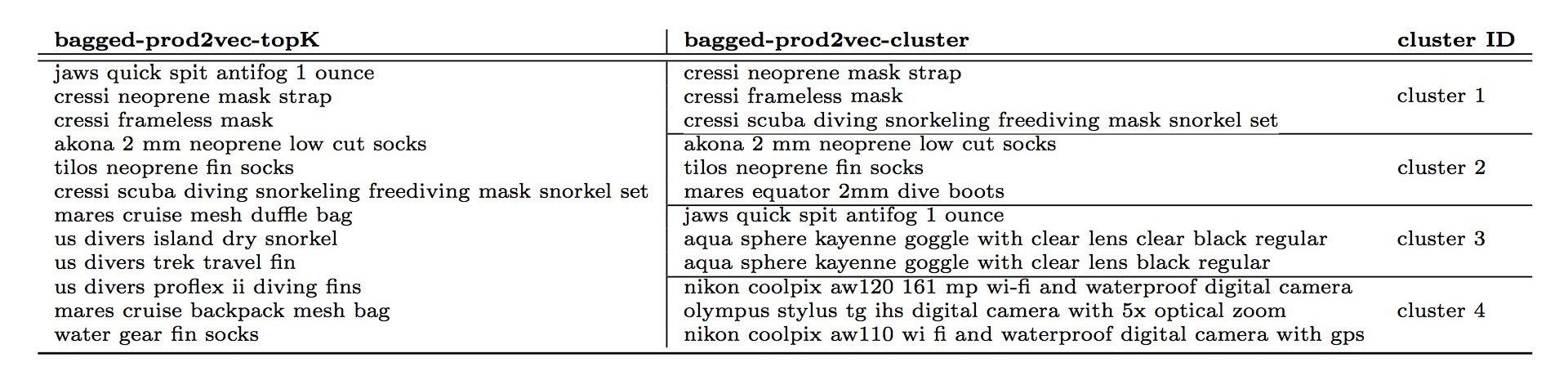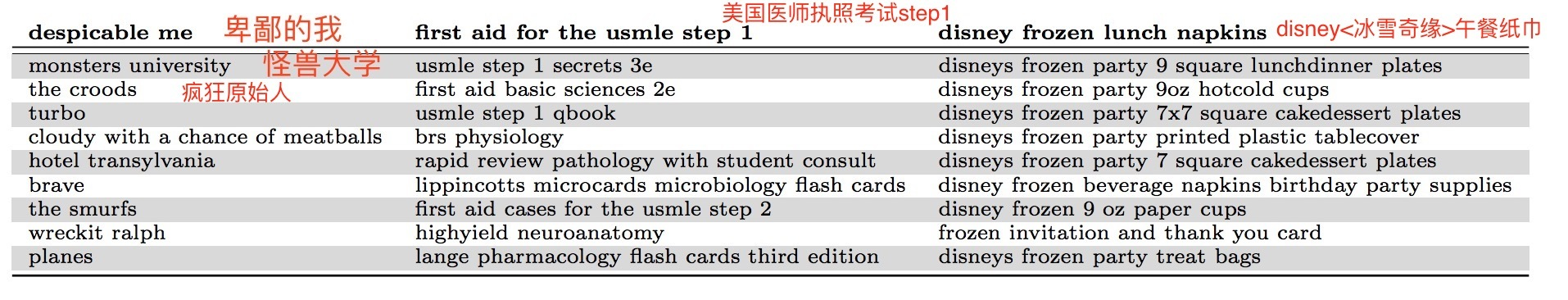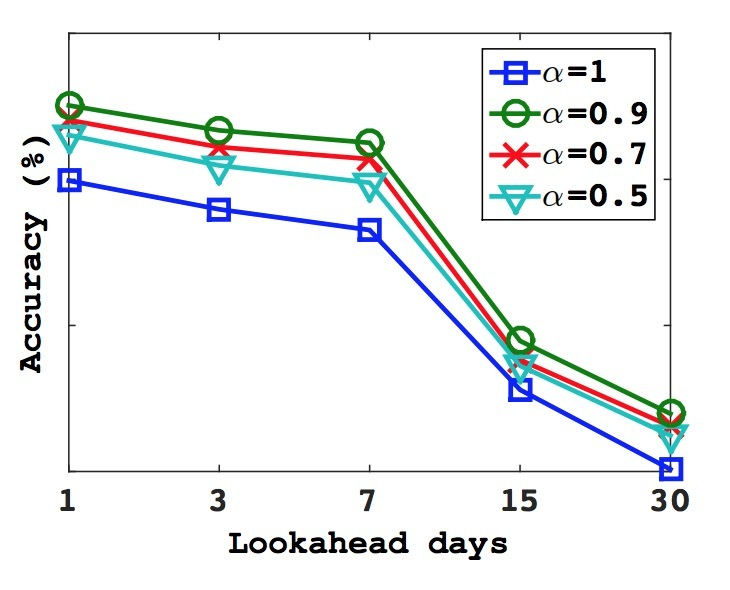Updated on